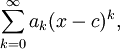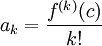# The Taylor Series

Previous: A Motivating Problem

Next: Maclaurin Expansion of ex

We have now found a method to calculate power series expansions for functions who have a certain property: that they have derivatives at all orders. Functions that have derivatives at all orders, on some open interval, are referred to as analytic on that interval. Functions that are analytic on an interval have what is called a Taylor series expansion.

Definition: The Taylor Series Expansion
Suppose that a given function, f(x), is analytic on an open interval that contains the point x = c. The Taylor series expansion for f(x) at c isand the coefficients of the series, ak are given byHere we are using the notation f(k) to denote the kth derivative of the given function, f(x).

The Taylor series obtained when we let c = 0 is referred to a Maclaurin series.

### When a Function Equals its Taylor Series

It is possible to show that if a given function is analytic on some interval, then it is equal to its Taylor series on that interval. That is, on an interval where f(x) is analytic,We will not prove this result here, but the proof can be found in most first year calculus texts. The proof involves

• a derivation for an expression for the difference, or remainder, between f and the Nth order partial sum of a power series expansion, and
• shows that if and only if the remainder goes to zero when N goes to infinity, the Taylor series converges to f(x).

There are functions that are not equal to its Taylor series expansion. But for the purposes of this module, we will assume that all functions can be expanded as a Taylor series.

Previous: A Motivating Problem

Next: Maclaurin Expansion of ex